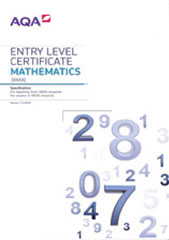# 3.2 Component 2: the four operations (calculator not allowed)

This content aims to develop the student’s understanding and use of the four operations.

Students will undertake calculations using mental arithmetic involving addition, subtraction, multiplication and division.

## Procedures for making and recording assessments

Class work assessed by the teacher in response to the outcomes set out below, or assessed by an externally-set assignment.

## Outcomes to be accredited

In successfully completing this component, students will have demonstrated the ability to:

### Entry 1

Outcomes

Notes/examples

GCSE reference

1.1 Add two whole numbers with a total up to 20

Zero can be one of the numbers

Key words are add, sum, total, altogether

Use the = sign to represent equality

N2

1.2 Subtract one number up to 20 from another

Zero can be the number subtracted

Key words are take, take away, subtract, difference, how much more, how much less

N2

1.3 Understand and use the + and – signs to solve simple number problems

Work out 5 + 11

6 = 10 - ?

Understand that subtraction is the inverse of addition

N2, N3

### Entry 2

Outcomes

Notes/examples

GCSE reference

2.1 Add whole numbers with a total up to 100

N2

2.2 Subtract one number up to 100 from another

N2

2.3 Multiply using single digit whole numbers

Key words are multiply, multiplication, times … lots of

Understand that multiplication is the same as repeated addition

N2

2.4 Use and interpret +, -, × and = in real-life situations for solving problems

N2

2.5 Recall and use multiplication facts for the 2, 5 and 10 multiplication tables

### Entry 3

Outcomes

Notes/examples

GCSE reference

3.1 Add and subtract using three digit numbers

N2

3.2 Multiply a two digit whole number by a single digit whole number

N2

3.3 Divide a two digit whole number by a single digit whole number

Key words are divide, division, divided by, share equally, equal groups of’

Understand that division is the same as repeated subtraction

Understand that division is the inverse of multiplication

Interpret the remainder in a practical situation

N2,N3

3.4 Use and interpret +, –, x ,÷ and = in real-life situations for solving problems

Could be multi-step

Kim bought three packs of 12 eggs. She used 4 eggs on Monday and 5 eggs on Tuesday. How many eggs did she have left?

N2

3.5 Use inverse operations to find missing numbers

Work out the missing number

35 + ? = 124

3.6 Estimate the answer to a calculation

3.7 Recall and use multiplication facts for the 3, 4 and 8 multiplication tables